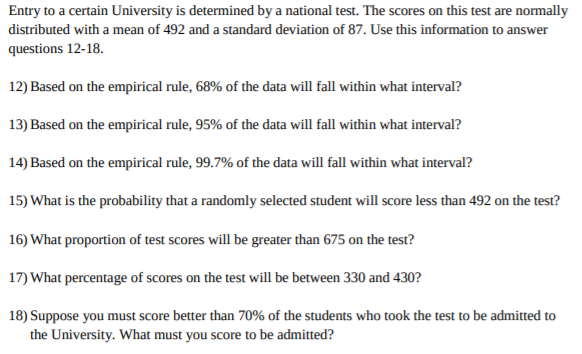# Entry to a certain University is determined by a national test. The scores on this test are normally distributed with a mean of 492 and a standard deviation of 87. Use this information to answer questions 12-18. 12) Based on the empirical rule, 68% of the data will fall within what interval? 13) Based on the empirical rule, 95% of the data will fall within what interval? 14) Based on the empirical rule, 99.7% of the data will fall within what interval? 15) What is the probability that a randomly selected student will score less than 492 on the test? 16) What proportion of test scores will be greater than 675 on the test? 17) What percentage of scores on the test will be between 330 and 430? 18) Suppose you must score better than 70% of the students who took the test to be admitted to the University. What must you score to be admitted?

Question

Need answers to questions 15 -18.help_outlineImage TranscriptioncloseEntry to a certain University is determined by a national test. The scores on this test are normally distributed with a mean of 492 and a standard deviation of 87. Use this information to answer questions 12-18. 12) Based on the empirical rule, 68% of the data will fall within what interval? 13) Based on the empirical rule, 95% of the data will fall within what interval? 14) Based on the empirical rule, 99.7% of the data will fall within what interval? 15) What is the probability that a randomly selected student will score less than 492 on the test? 16) What proportion of test scores will be greater than 675 on the test? 17) What percentage of scores on the test will be between 330 and 430? 18) Suppose you must score better than 70% of the students who took the test to be admitted to the University. What must you score to be admitted? fullscreen

### Want to see this answer and more?

Experts are waiting 24/7 to provide step-by-step solutions in as fast as 30 minutes!*